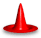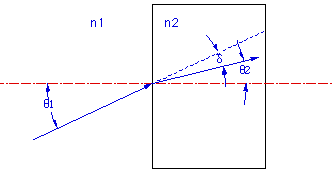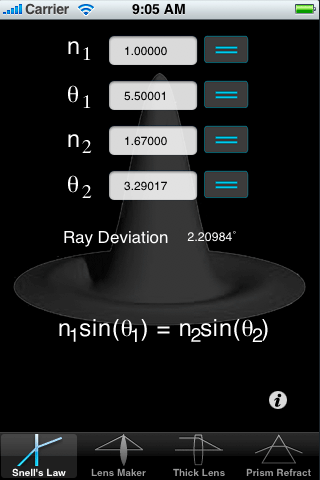OpticsCalc for iPhone: Snell's LawDescriptionThe Snell's Law page computes the refraction at the boudary between two materials of different indices n1 and n2. The incident ray in material 1 is incident on the interface at angle θ1 with respect to the surface normal. The ray then refracts at the surface and enters material 2 at an angle of θ2 relative to the optical axis. The ray is deviated by a total angle shown as δ in the figure.

n1sin(θ1) = n2sin(θ2)This page allows you to enter any 3 of the 4 values and compute the fourth. After you fill in the known quantities, select the value you want to calculate by pressing the appropriate equal sign. Note that the ray deviation &delta is computed automatically.A Note On UnitsAll angles are in degrees.